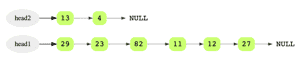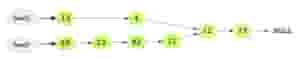# Intersection Point of Two Linked Lists

## Problem Statement

Given the head nodes of two linked lists that may or may not intersect, find out if they do in fact intersect and return the point of intersection. Return null otherwise.

In the below example, neither lists intersects. Intersect() should return NULL.After adding nodes 12 and 27 in linked list head2, the list now have two same nodes as the linked list head1.However, in the below example, both lists intersect at the node with data 12, so the node 4 in linked list head2 points to node 12 and the node 11 in linked list head1 points to node 12 (have same address).## Hint

• Find the length of both linked lists
• Use HashSet

## Try it yourself

LinkedListNode* intersect(
//TODO: Write - Your - Code
}

## Solution

static int get_length(LinkedListNode* head) {
int list_length = 0;
list_length++;
}
return list_length;
}

int length_difference = 0;
if(list1length >= list2length) {
length_difference = list1length - list2length;
} else {
length_difference = list2length - list1length;
}

while(length_difference > 0) {
list1node = list1node->next;
length_difference--;
}

while(list1node != nullptr) {
if(list1node == list2node) {
return list1node;
}
list1node = list1node->next;
list2node = list2node->next;
}
return nullptr;
}

int main(int argc, char* argv[]) {
vector<int> v1 = {1, 2, 3, 4 , 5};

vector<int> v2 = {5, 4, 3, 2, 1};

assert(intersect_node == nullptr);

printf("\nIntersection node: %d", intersect_node->data);
assert(intersect_node == node1);

assert(intersect(nullptr, nullptr) == nullptr);

}

## Solution Explanation

### Runtime Complexity

Linear, O(m + n).

Where m is the length of the first linked list and n is the length of the second linked list.

Constant, O(1).

### Solution Breakdown

The first solution that comes to mind is one with quadratic time complexity i.e. for each node in the first linked list, a linear scan must be done in the second linked list. If any node from the first linked list is found in the second linked list (comparing addresses of nodes, not their data), that is the intersection point. However, if none of the nodes from the first list are found in the second list, that means there is no intersection point.

Although this works, it is not efficient. A more efficient solution would be to store the nodes of the first linked list in a HashSet and then go through the second linked list nodes to check whether any of the nodes exist in the HashSet. This approach has a linear runtime complexity and linear space complexity.

Below is the complete algorithm.

• Find the lengths of both linked lists: L1 and L2
• Calculate difference in length of both linked lists: d = |L1 - L2|
• Move head pointer of longer list ‘d’ steps forward
• Now traverse both lists, comparing nodes until we find a match or reach the end of lists

Learn in-demand tech skills in half the time